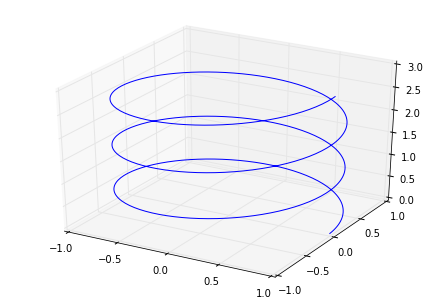## Problem 1

In this problem, we'll implement the famous Lorentz system.

\begin{align} \dot{x} &= \sigma (y - x) \\ \dot{y} &= x(\rho - z) - y \\ \dot{z} &= xy - \beta z \end{align}

We'll try this for a few different choices of $\sigma$, $\rho$ and $\beta$.

First, try computing a solution using odeint with parameters $\sigma = 10, \rho = 28, \beta = 8/3$.

Since there are three variables now, try plotting the various projections to the $(x,y)$-plane, $(x,z)$-plane and $(y,z)$-plane.

### Bonus: Plotting in 3D

Sometimes there really is a need to do a 3D plot...and it's just pretty cool to be able to do. This bonus section will introduce you to one of Matplotlib's "toolkits". These are various add-ons to the standard Matplotlib library which let you do some interesting things. To access the 3D toolkit, we'll use the following code snippit:

In :
%matplotlib inline
import matplotlib.pyplot as plt
from mpl_toolkits.mplot3d import Axes3D


To actually do the 3D plotting, we'll make a slight change to our plotting code. For example, if I want to plot a helix in 3D, I can use:

In :
t = np.linspace(0, 3, 500)
x = np.cos(2*np.pi*t)
y = np.sin(2*np.pi*t)
z = t

fig = plt.figure()
ax = Axes3D(fig) # <- sets up a 3D plotting object
ax.plot(x, y, z) # <- notice that now we can give it (x, y, z) data
plt.show()## A Different Equation and Method: Challenge

This problem is intended for people who have seen PDEs and tools like the Fourier transform and who are interested.

Consider the PDE $u_t + u_{xxx} = 0$ on a periodic domain. This time we'll take an entirely different approach by using the Fourier transform. We know that by applying the Fourier transform to our equation, we get:

$$\hat{u}_t - i \xi^3 \hat{u} = 0$$

Since this is just the an ODE, we can solve it as:

$$\hat{u} = e^{i\xi^3t} \hat{u}_0$$

Finally, we can compute the inverse Fourier transform to get the solution in the time domain. Now, this last step leads to a total different idea for a method. What we'll do is try using a Fast Fourier transform on our data to approximate solving the problem this way. The main steps along the way will look something like:

In :
# 0. Import fft tools we'll need.
from scipy.fftpack import fft, ifft, fftfreq

# 1. Set up the corresponding x / xi coordinates.
n = 32
x = np.linspace(0, 1, n)
xi = fftfreq(n, d=x-x) # <- d= is just the mesh spacing.

# 2. After constructing your initial data u0, construct u0 hat. For example, a step.
u0 = np.where((.4 < x) & (x < .6), 1.0, 0.0)
u0hat = fft(u0)

# 3. For various values of t, compute u hat
t = 1.4
uhat = np.exp(1.0j * xi**3 * t) * u0hat

# 4. To get a solution in the original coordinates, compute the inverse fft
u = ifft(uhat)


### A Different, Different Equation

One equation which has been very well studied is the KdV equation. I won't ask you to implement this, but one method used to simulate this uses a combination of what we've seen for ODEs and the method introduced above. You can read more about it here if you're interested.